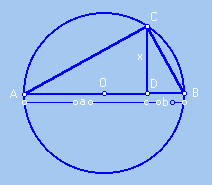### Construction of a square with area the same as that of a rectangle with side a and b.

Given two segments of lengths  a and b, to construct the segment with  length equal to the square root of a.b, we proceed as follows:
1. Line up the two segments of length and b on a single line.
2. Trace the circle of center in the midpoint of the lined up segments.
3. At the common extremity D to the two segments draw a perpendicular segment to the diameter, meeting the circle at the point C.The segment CD has as length the square root of a.b. In fact, the similarity between triangles ACD and CBD (both of which are similar to the ABC triangle) we have that:  AD/CD = BD/CD, or by "cross multiplying" CD.CD = AD.BD  or either, the length CD  is the square root of the product of the lengths of CA and CB.  The square on the side CD has the same area as the rectangle with sides a and b.